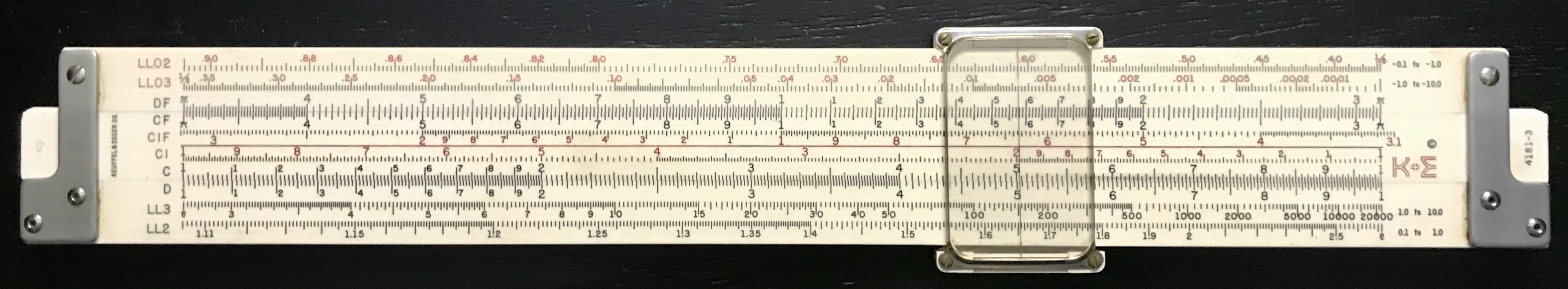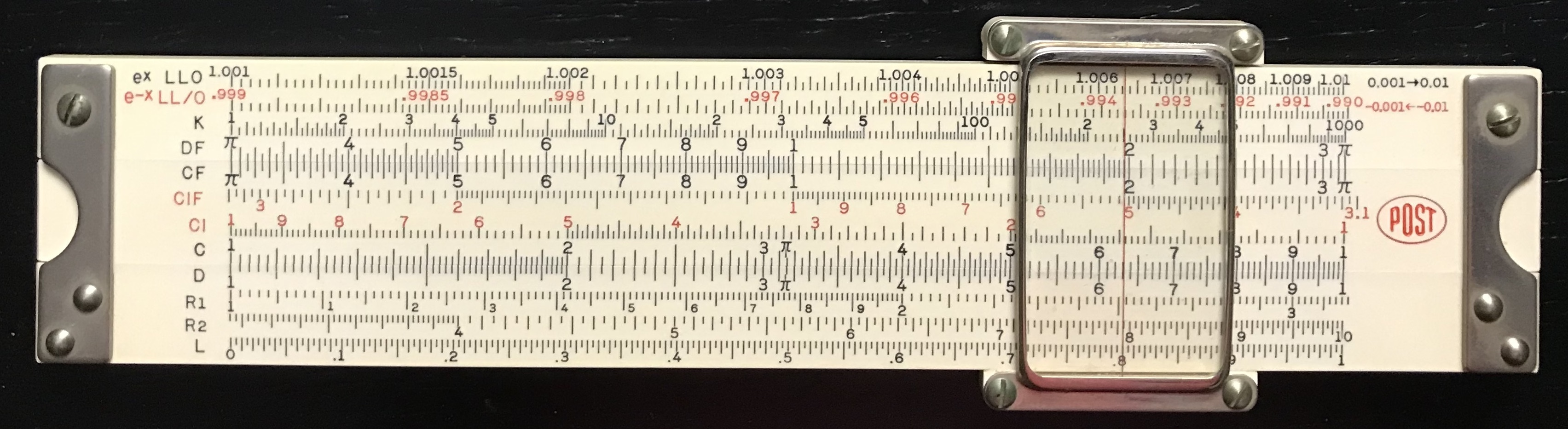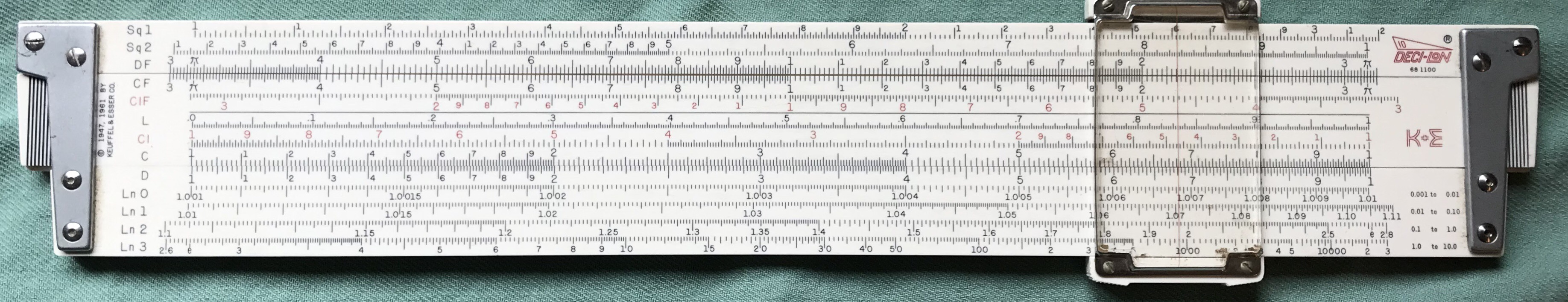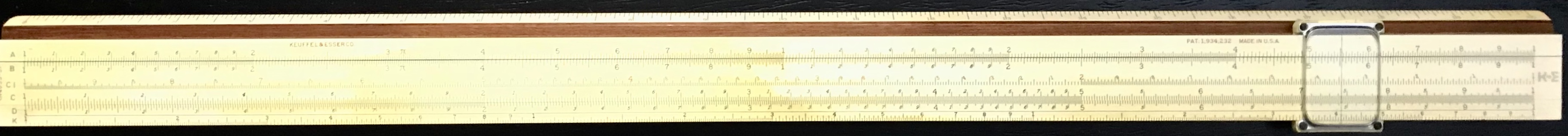## 9.12 The Long and Short of It

Suppose we have a slide rule whose main C/D scales are of length $$L_0$$. Let’s make an estimate of the effect that $$L_0$$ has on the accuracy of the result of a calculation performed on the rule. The actual distance $$L$$ from the left-hand side of the scale (“1”) to a marking corresponding to value $$x$$ on the scale is intended to be given by $$L = L_0 \log x$$. Re-written in terms of the natural logarithm, this is

$L = L_0 \log x = \frac{L_0}{\ln 10}\; \ln x.$

Since the derivative of the natural logarithm with respect to $$x$$ is $$\frac{d}{dx}(\ln x) = 1/x$$, then differentiating the above equation we find that $dL = \frac{L_0}{\ln 10} \cdot \frac{1}{x} dx ~~ \approx ~~ \frac{L_0}{2} \cdot \frac{dx}{x}.$This tells us that for a slide rule with C/D scales of length $$L_0$$ and with an error in the marking/setting/reading of the rule $$\Delta L$$, the relative error in the numerical result, $$\Delta x/x$$, will be approximately

$\frac{\Delta x}{x} \approx \frac{2\Delta L}{L_0}.$

Now if the error $$\Delta L$$ in the rule marking/setting is independent of the length of the rule, then the relative error of the result $$\Delta x/x$$ will scale inversely with the length of the slide rule, $$L_0$$. For example, if one had a 25 cm slide rule, then typical settings/readings accuracies of about $$\Delta L \approx$$ 0.25 mm would give a relative accuracy in the result of about 2 $$\times$$ 0.25 mm / 250 mm = 0.2%. The longer the slide rule scales, the higher the accuracy of the results.

The standard slide rule scale length of 10 inches, or 25 cm, was no doubt a relic of the standard 12-inch, or 1 foot, measuring rule and gave sufficient – roughly 3-digit – accuracy in most computations. And, of course, long-scale rules were available for more accuracy. But pocket versions of the more popular slide rules were quite common in the middle of the 20th century. While an engineer might have a 10-inch slide rule on the desk back in the office, and perhaps a 20-inch slide rule in the cabinet for getting that last digit correct for a publication result, a smaller pocket version with identical scales could be invaluable for the person working “in the field” during much of the work day, where quick calculations might be needed on the spot.The popular Versalog (Post/Hemmi) and Deci-Lon (K&E) 10-inch slide rules found in the Collection had corresponding 5-inch, or “pocket” versions with the exact same scale sets. Another example from the Collection is the K&E Model 4181-3 (10-inch) and Model 4181-1 (5-inch), also popular since the 1950’s. Many other similar pairs can be found from many manufacturers.

Collection Photos 10-in. model 5-in. model
K&E 4181 c. 1957Versalog c. 1965Deci-Lon c. 1970The 10-inch and 5-inch “pocket” lengths became fairly standard by the mid-1900’s, but K&E produced several of its models with even more variety in terms of length well before that time. For example, the popular K&E 4053 Polyphase slide rule was introduced as a 10-inch rule in 1909. By 1911, the rule was sold in three different lengths, differentiated by the use of a dash at the end of the model number:37

4053 Collection Photos
-5 50 cm c. 1937-3 25 cm c. 1925-2 20 cm c. 1910The number after the dash indicates the approximate length in decimeters (dm = 10 cm): -5 for 5 dm, -3 for 2.5 dm, and -2 for 2 dm. The 4053 family of rules was the first to use this designation in the K&E line-up.

Looking through the K&E catalogs, one might believe that the 4083 family had three different lengths as well. However, while the original 4083 (no dash) was 16 inches in length, first produced in about 1906 as a Polyphase Duplex rule, this model was discontinued and later re-issued – in 1939 – for the new vector slide rules, models 4083-3 and 4083-5, which had a completely different set of scales.

The only other K&E model line that had more than a couple of available lengths was the Model 4088. This slide rule family was introduced in 1913. In this year, Models 4088-2 and 4088-3 were made available, with the 4088-5 coming out in 1916.38 Then, in 1922, a shorter “pocket” model – the 4088-1 – was introduced. Also in that year, a B scale was added to all of the models of this family. With the new 4088-1, the K&E 4088 family was the only one with four different lengths available for sale simultaneously. These rules were produced until 1939, at which time the Model 4071-3 replaced them, other lengths of this model being discontinued. Below is a sample of the 4088 family of rules found in the Collection: